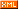# 朱燚

--书到读透处，酒于微醺时

 博客园 :: 首页 :: 博问 :: 闪存 :: 新随笔 :: 联系 :: 订阅:: 管理 ::.Net提供了许多多线程编程工具,可能是因为太多了,所以掌握起来总是有一些头疼,我在这里讲讲我总结的一些多线程编程的经验,希望对大家有帮助

ThreadStart threadStart=new ThreadStart(Calculate);

public void Calculate(){
double Diameter=0.5;
Console.Write("The perimeter Of Circle with a Diameter of {0} is {1}"Diameter,Diameter*Math.PI);
}





public void Calculate(object arg){
double Diameter=double(arg);
Console.Write("The perimeter Of Circle with a Diameter of {0} is {1}"Diameter,Diameter*Math.PI);
}



Calculate方法有一个为object类型的参数,虽然只有一个参数,而且还是object类型的,使用的时候尚需要类型转换,但是好在可以有参数了,并且通过把多个参数组合到一个类中,然后把这个类的实例作为参数传递,就可以实现多个参数传递

public class MyThread
{
public double Diameter=10;

public double Result=0;

{
this.Diameter = Diameter;
}

public void Calculate()
{
Console.WriteLine( "Calculate Start");
Result = Diameter*Math.PI;;
Console.WriteLine("Calculate End, Diameter is {0},Result is {1}" ,this.Diameter, Result);
}
}



double Diameter = 6;

double Result=0;
{

Result=Diameter * Math.PI;
Console.WriteLine("匿名 Calculate  End, Diameter is {0},Result is {1}", Diameter, Result); ;
}));
ta.Start();



delegate double CalculateMethod(double Diameter);

static CalculateMethod calcMethod;
double result = 0;

static void Main(string[] args)
{

calcMethod = new CalculateMethod(Calculate);

}

///<summary>
///线程调用的函数
///<summary>

public static double Calculate(double Diameter)
{
return Diameter * Math.PI;
}

///<summary>
///线程完成之后回调的函数
///<summary>
{
result=calcMethod.EndInvoke(result);
}



WaitCallback w = new WaitCallback(Calculate);

public static void Calculate(double Diameter)
{
return Diameter * Math.PI;
}



[注]由于每个进程只有一个线程池,所以如果是在iis进程,或者sqlserver的进程中使用线程池,并且需要设置线程池的最大容量的话,会影响到iis进程或sql进程,所以这两种情况下要特别小心

public static void TaskFinished(IAsyncResult result)
{
result=calcMethod.EndInvoke(result);
this.TextBox1.Text=result;
}


delegate void changeText(string result);